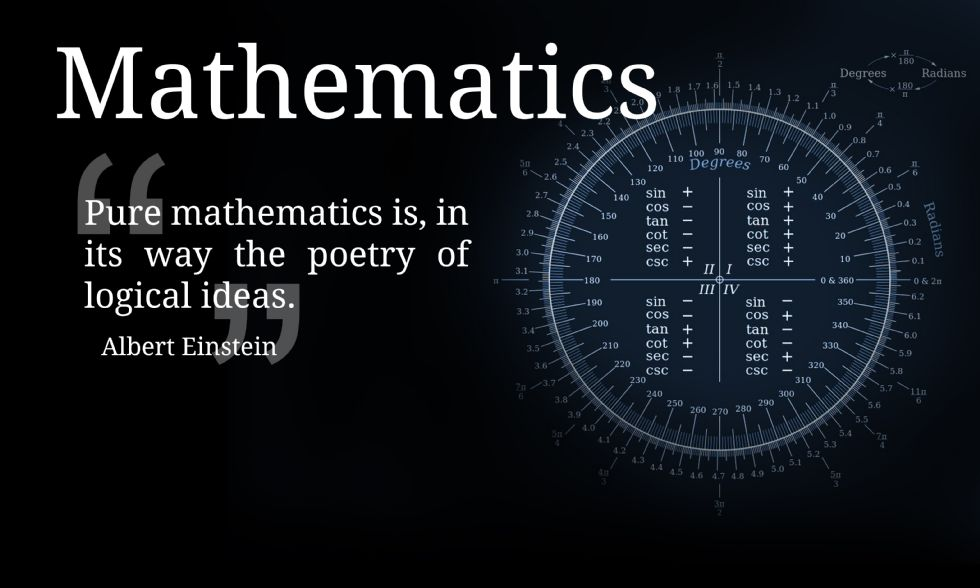• Home Learning

Please remember that online learning from home resumes on Monday 20th April. Students will need to login to 'Teams' for details.

Water Orton Road, Castle Bromwich, Birmingham. B36 9HF

Telephone:0121 748 0400

Fax:0121 748 0414

Contact:Mr Cox

# Year 10### Year 10 Foundation

 Assessment Cycle Topics covered 1 Four operations BIDMAS Place Value Rounding and estimation Squares, cubes, roots and other powers Index laws Factors, multiples and primes HCF and LCM Simplifying algebra Expanding and factorising single brackets Substitution Forming expressions Data collection sheets Two way tables Time series Time Coordinates 2 Pictograms Interpreting frequency tables Draw and interpret pie charts Scatter graphs Fractions, decimals and percentages Add and subtract fractions Multiply and divide fractions Percentage of amount Percentage increase and decrease Forming and solving equations Rearrange equations Inequalities Representing inequalities pictorially Solving inequalities 3 Sequences Nth term of a sequence Types of data Averages Averages from a frequency table Drawing angles Angle rules Angles in parallel lines Angles in polygons Reading scales Converting units Area and perimeter Surface area Nets Plotting linear graphs Equation of a straight line graph Summer Reading and interpreting straight line graphs Distance-time/Velocity-time graphs Transformations Congruency and similarity

### Year 10 Higher

 Assessment Cycle Topics covered 1 Four operations Rounding and estimation Index laws, including fractional and negative Factors, multiples and primes HCF and LCM Standard form Simplifying surds Substitution Expanding and factorising single brackets Expanding and factorising quadratics Form and solve equations Change the subject of a formula Iteration Proof Linear sequences Nth term Quadratic sequences Nth term of a quadratic sequence Arithmetic and geometric sequences Two way tables Averages Averages from a frequency table, both grouped and discrete 2 Draw and interpret pie charts Time-series graphs Frequency polygons Scatter graphs Fractions, decimals and percentages Add,  subtract, multiply and divide fractions Recurring decimals Percentage of an amount Percentage increase and decrease Compound interest Reverse percentages Sharing in a given ratio Representing ratio as a fraction Recipes Conversion graphs Angle rules Angles in parallel lines Angles ion polygons Pythagoras’ Theorem Trigonometry 3 Coordinates Distance-time/Velocity-time graphs Midpoints of line segments Length of line segments Equation of a line segment Plotting linear graphs Equation of a straight line Parallel and perpendicular lines Plotting quadratics, cubics and reciprocal graphs Area and circumference of circles Area and perimeter of sectors Volumes of prisms Surface area of prisms Volume of non-prisms Surface area of non-prisms Upper and lower bounds Calculations involving upper and lower bounds Summer Transformations Loci and constructions